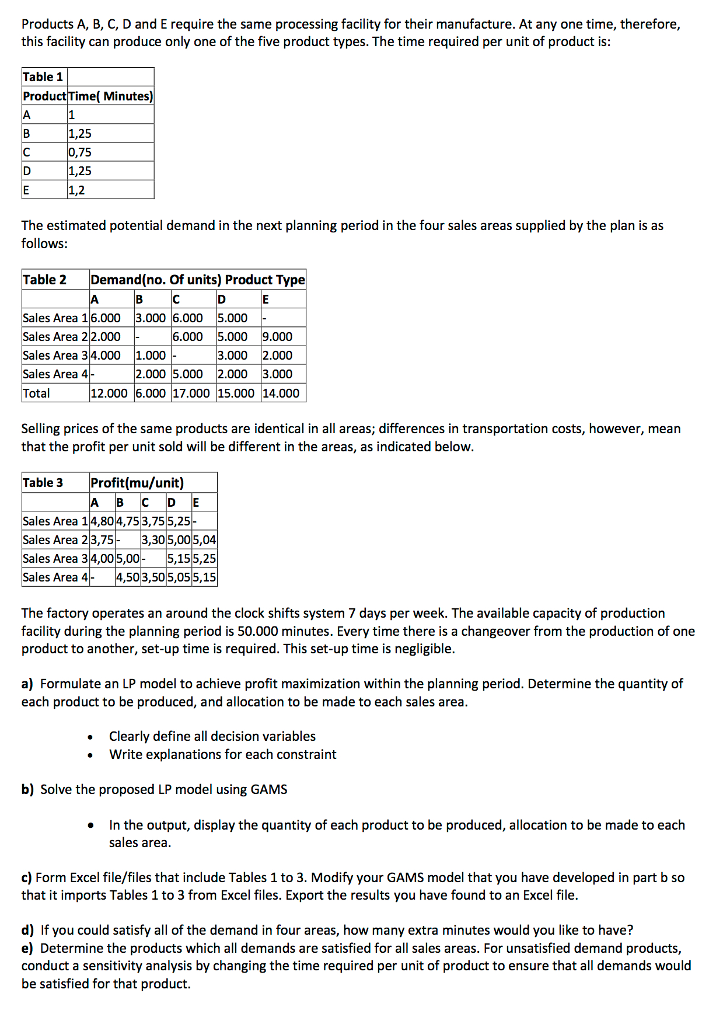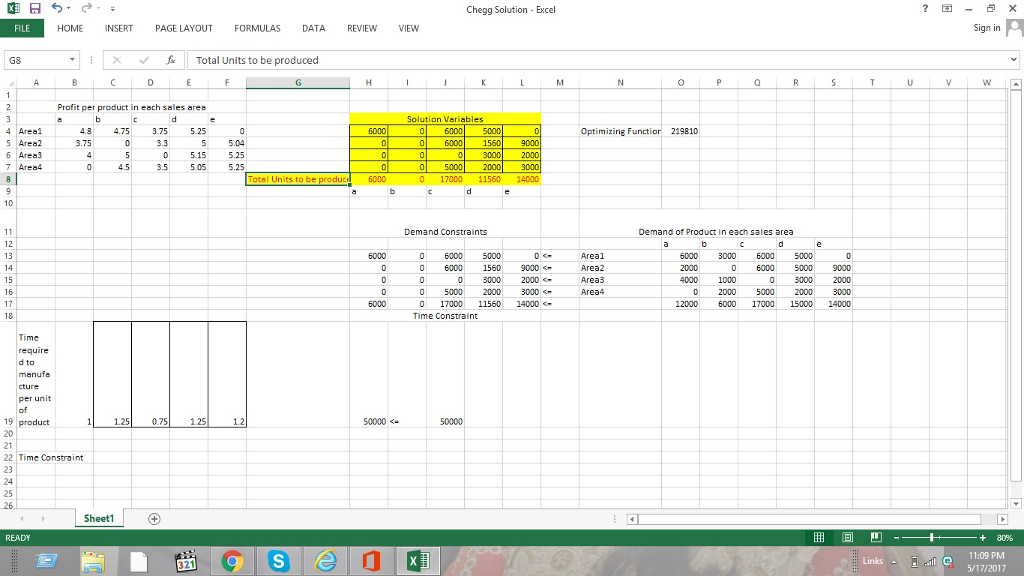# Question & Answer: The question is about GAMS programming language which is used in linear programming methods. So that, the…..

The question is about GAMS programming language which is used in linear programming methods. So that, the question should be coded in GAMS language. Asking for writing this problem in proper code.Let a1 units of Product A be sold in Sales Area 1

Let a2 units of Product A be sold in Sales Area 2

Don't use plagiarized sources. Get Your Custom Essay on
Question & Answer: The question is about GAMS programming language which is used in linear programming methods. So that, the…..
GET AN ESSAY WRITTEN FOR YOU FROM AS LOW AS \$13/PAGE

Let a3 units of Product A be sold in Sales Area 3

Let a4 units of Product A be sold in Sales Area 4

Let b1 units of Product B be sold in Sales Area 1

Let b2 units of Product B be sold in Sales Area 2

Let b3 units of Product B be sold in Sales Area 3

Let b4 units of Product B be sold in Sales Area 4

Let c1 units of Product C be sold in Sales Area 1

Let c2 units of Product C be sold in Sales Area 2

Let c3 units of Product C be sold in Sales Area 3

Let c4 units of Product C be sold in Sales Area 4

Let d1 units of Product D be sold in Sales Area 1

Let d2 units of Product D be sold in Sales Area 2

Let d3 units of Product D be sold in Sales Area 3

Let d4 units of Product D be sold in Sales Area 4

Let e1 units of Product E be sold in Sales Area 1

Let e2 units of Product E be sold in Sales Area 2

Let e3 units of Product E be sold in Sales Area 3

Let e4 units of Product E be sold in Sales Area 4

Our objective function is to maximize profit i.e.

Maximize Z = 4.8a1 + 3.75a2 + 4a3 + 4.75b1 + 5b3 + 4.5b4 + 3.75c1 + 3.3c2 +3.5c4 + 5.25d1 + 5d2 +5.15d3 + 5.05d4 + 5.04e2 + 5.25e3 +5.15e4

Now applying the time constraints:

1(a1 + a2 + a3) + 1.25(b1 + b3 + b4) + 0.75(c1 + c2 + c4) +1.25(d1 +d2 + d3 + d4) + 1.2(e2 + e3 + e4) <= 50000

Demand Constraints

Production of each product should be less than or equal to the sum of demand in all Sales Area.

a1 + a2 + a3 < =12000

b1 + b3 + b4<= 6000

c1 + c2 + c4 <= 17000

d1 +d2 + d3 + d4<= 15000

e2 + e3 + e4 <=14000

Supply for the product in each area should be less than or equal to the demand for that product in that area

a1< =6000 , a2< =2000, a3 < = 4000

b1 <= 3000,  b3 <= 1000 ,  b4 <= 2000

c1 <=6000 c2 < =6000, c4 < =5000

d1<=5000,   d2<=5000,   d3<=3000,   d4<=2000

e2 <= 9000,  e3< =2000,  e4 <= 3000

Finally the time constraint,

The time required for production should be less than or equal to 50000

i.e. (Time required per unit production of product * Number of Units) for all products <= 50000

1(a1+a2+a3+a4) + 1.25(b1+b2+b3+b4) + 0.75(c1+c2+c3+c4) + 1.25(d1+d2+d3+d4) + 1.2(e1+e2+e3+e4) < =50000Solved this problem using excel solver. Please check the image with answer. Let me know if you require the spreadsheet.

Optimal Solution is:

 Product Number of Units A 6000 B 0 C 17000 D 11560 E 14000